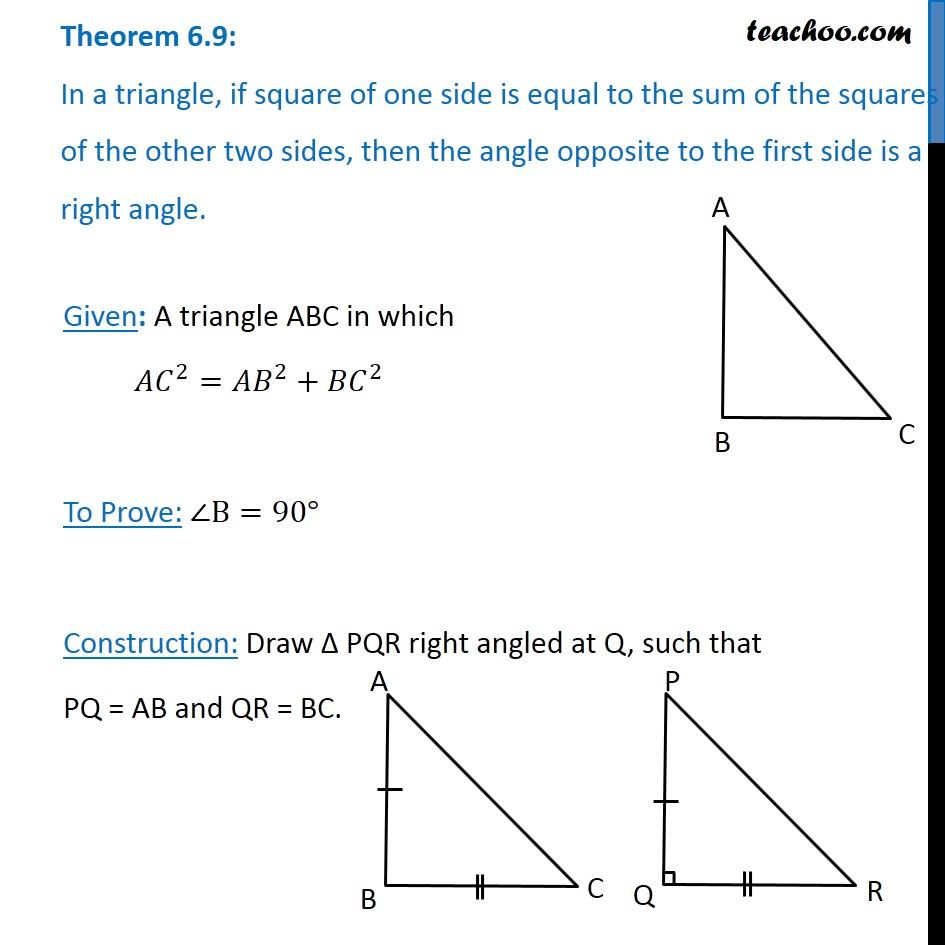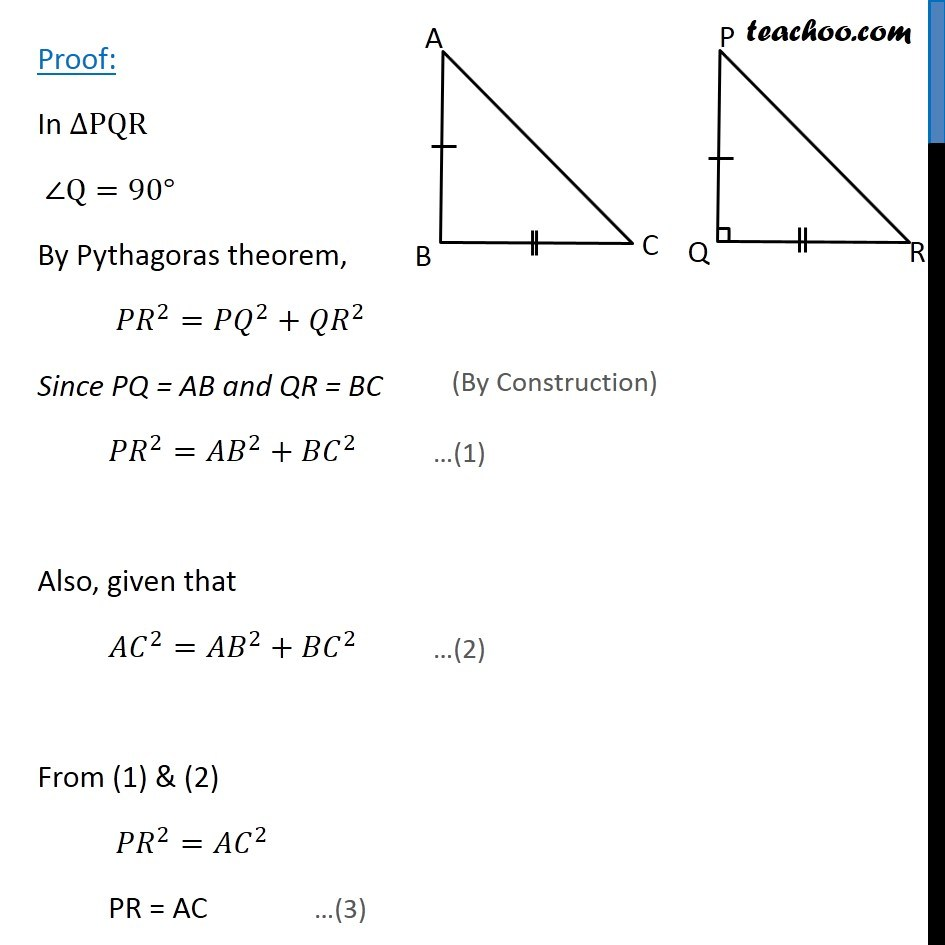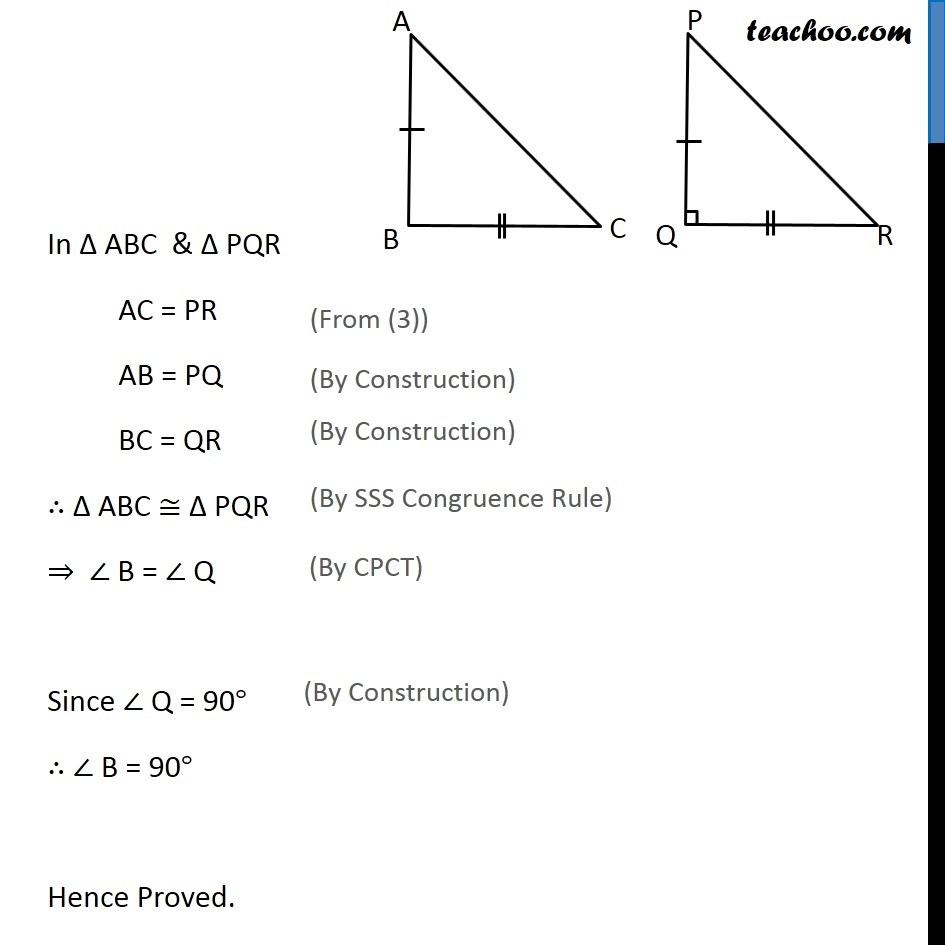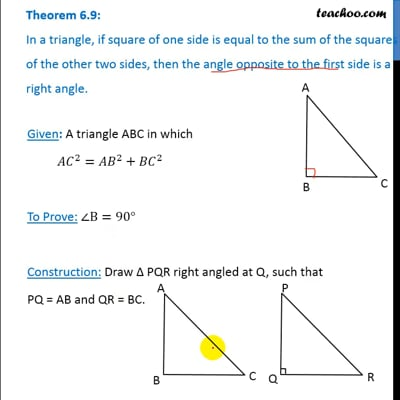Theorems

Chapter 6 Class 10 Triangles (Term 1)
Serial order wiseThis video is only available for Teachoo black users

### Transcript

Theorem 6.9: In a triangle, if square of one side is equal to the sum of the squares of the other two sides, then the angle opposite to the first side is a right angle. Given: A triangle ABC in which 〖𝐴𝐶〗^2=〖𝐴𝐵〗^2+〖𝐵𝐶〗^2 To Prove: ∠B=90° Construction: Draw Δ PQR right angled at Q, such that PQ = AB and QR = BC. Proof: In ∆PQR ∠Q=90° By Pythagoras theorem, 〖𝑃𝑅〗^2=〖𝑃𝑄〗^2+〖𝑄𝑅〗^2 Since PQ = AB and QR = BC 〖𝑃𝑅〗^2=〖𝐴𝐵〗^2+〖𝐵𝐶〗^2 Also, given that 〖𝐴𝐶〗^2=〖𝐴𝐵〗^2+〖𝐵𝐶〗^2 From (1) & (2) 〖𝑃𝑅〗^2=〖𝐴𝐶〗^2 PR = AC In Δ ABC & Δ PQR AC = PR AB = PQ BC = QR ∴ Δ ABC ≅ Δ PQR ⇒ ∠ B = ∠ Q Since ∠ Q = 90° ∴ ∠ B = 90° Hence Proved.